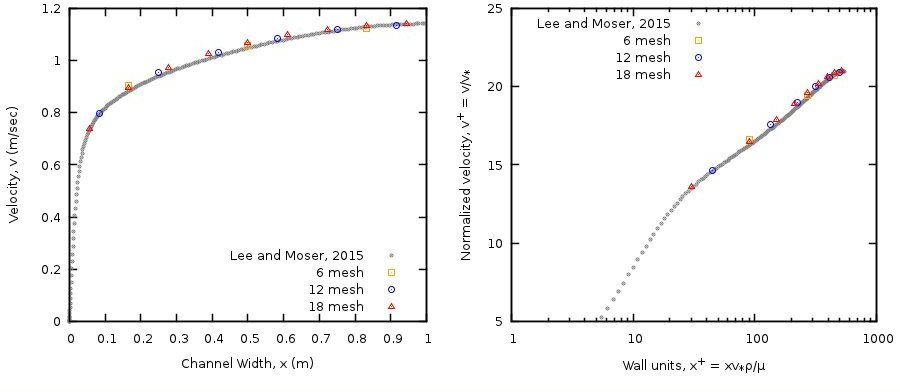# 3.7. FLD07: Steady, 2D fully-developed, turbulent channel flow¶

## 3.7.1. Description¶

This case uses 2D, fully-developed turbulent channel flow between two horizontal, parallel plates separated by a width, $$W$$, to assess the single phase k-ϵ model in MFIX. Periodic boundaries with a specified pressure drop are imposed in the y-direction as shown in Fig. 3.18.Fig. 3.18 Turbulent flow in a 2D channel

The pressure drop along the channel is equated to the shear stress at the walls, $$\tau_{w}$$.

(3.11)$W\frac{dP_{g}}{\text{dy}} = {2\tau}_{w}$

The shear stress is related to the gas density, $$\rho_{g}$$, and friction velocity, $$v_{*}$$,

(3.12)$\tau_{w} = \rho_{g}v_{*}^{2},$

where, the friction velocity, is given by the Reynolds number.

(3.13)$\text{Re}_{\tau} = \frac{\rho_{g}v_{*}(W/2)}{\mu_{g}}$

## 3.7.2. Setup¶

#########################################################################
#                                                                       #
# Author: Avinash Vaidheeswaran                         Date: July 2016 #
# Turbulent flow in a pipe problem:                                     #
#                                                                       #
# Turbulent flow through a channel is simulated and the results are     #
# compared with the data from DNS                                       #
#                                                                       #
#########################################################################

RUN_NAME = 'FLD07'
DESCRIPTION = 'Turbulent channel flow'

#_______________________________________________________________________
# RUN CONTROL SECTION

UNITS = 'SI'
RUN_TYPE = 'NEW'

TSTOP = 1.0d8

DT = 0.02

ENERGY_EQ =     .F.
SPECIES_EQ(0) = .F.

GRAVITY = 0.0

CALL_USR = .T.

#_______________________________________________________________________
# NUMERICAL SECTION

DISCRETIZE(1:9) = 9*2

NORM_g = 0.0

#_______________________________________________________________________
# GEOMETRY SECTION

COORDINATES = 'CARTESIAN'

ZLENGTH =  1.00     NO_K = .T.
XLENGTH =  2.00     IMAX =  8
YLENGTH =  1.00     JMAX =  4

#_______________________________________________________________________
# GAS-PHASE SECTION

RO_g0 = 1.0        ! (kg/m3)
MU_g0 = 1.0d-04    ! (Pa.s)

TURBULENCE_MODEL = 'K_EPSILON'

MU_GMAX =  1.0d6   ! (Pa.s)

#_______________________________________________________________________
# SOLIDS-PHASE SECTION

MMAX = 0

#_______________________________________________________________________
# INITIAL CONDITIONS SECTION

IC_X_w(1) =      0.0     ! (m)
IC_X_e(1) =      2.0     ! (m)
IC_Y_s(1) =      0.0     ! (m)
IC_Y_n(1) =      1.0     ! (m)

IC_EP_G(1) =     1.0

IC_P_G(1) =      0.0     ! (Pa)

IC_U_G(1) =      1.0d-6  ! (m/sec)
IC_V_G(1) =      1.0     ! (m/sec)

IC_K_TURB_G(1) = 0.010   ! (m2/s2)
IC_E_TURB_G(1) = 0.001   ! (m2/s3)

#_______________________________________________________________________
# BOUNDARY CONDITIONS SECTION

! Flow boundaries: Periodic with specified pressure drop
!---------------------------------------------------------------------//
CYCLIC_Y_PD = .T.
DELP_Y = @(0.0543496*0.0543496)    ! (Pa)

! The east and west boundaries are no-slip walls (NSW)
!---------------------------------------------------------------------//

BC_X_w(1:2) =     0.0     2.0    ! (m)
BC_X_e(1:2) =     0.0     2.0    ! (m)
BC_Y_s(1:2) =     0.0     0.0    ! (m)
BC_Y_n(1:2) =     1.0     1.0    ! (m)

BC_TYPE(1:2) = 2*'NSW'

#_______________________________________________________________________
# OUTPUT CONTROL SECTION

RES_DT = 1.0d6
SPX_DT(1:9) = 9*1.0

FULL_LOG = .F.
RESID_STRING  =    'P0' 'U0' 'V0' 'K0'

#_______________________________________________________________________
# DMP SETUP

!  NODESI = 1    NODESJ = 2    NODESK = 1


## 3.7.3. Results¶

The pressure drop in the y-axial direction, domain length and width, and gas density were chosen to reflect the conditions of Lee and Moser  for $$\text{Re}_{\tau} = 543$$. The DNS dataset was accessed on November 10, 2016 from http://turbulence.ices.utexas.edu/channel2015/data/LM_Channel_0550_mean_prof.dat.

Transient simulations were performed for better numerical stability. The solution was considered converged when the L2 norms for the gas velocity components, $$u_{g}$$ and $$v_{g}$$, turbulent kinetic energy, $$k_{g}$$, and rate of turbulent kinetic energy dissipation, $$\epsilon_{g}$$, were all less than 10-10.

Simulations were conducted for three mesh levels [6, 12, 18] in the x-axial direction. Mesh levels were selected to ensure that the stream-ways velocity components in computational cells adjacent to the wall were located outside the buffer layer. Specifically, the first stream-ways velocity component should be located at least 30 wall units from the wall to be consistent with the $$k - \epsilon$$ model wall function implementation.

(3.14)$\frac{\Delta x}{2}\frac{\ v_{*}\rho_{g}}{\mu_{g}} > 30$

The MFIX results are shown in Fig. 3.19 along with the direct numerical simulation (DNS) data of Lee and Moser  for $$\text{Re}_{\tau} = 543$$. The velocity profiles for the three mesh levels are shown on the left whereas the normalized velocity profiles with respect to wall units are shown on the right.Fig. 3.19 2D, fully developed, turbulent channel flow with the DNS data of Lee and Moser  ; (Left) Velocity profile; (Right) Non-dimensionalized channel width and velocity profile.